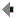Book Contents

Ch. 9
Building Model-Driven Decision Support Systems# Break-Even AnalysisFigure 9.1 Break-even DSS developed in Excel.

A break-even calculation shows the level of operations in units produced at which revenues just cover costs (profit equals zero). The break-even volume can be computed in a number of ways. One approach divides fixed costs by the contribution margin to find the break-even quantity. The contribution margin is the selling price per unit minus the variable costs per unit. Also, the breakeven quantity can be calculated by solving the expression: (Price * Quantity Sold) - (Fixed Cost + (Variable Cost per unit * Quantity Sold)) = 0.

A typical break-even model assumes a specific fixed cost and a constant average variable cost. The break-even quantity can be calculated in a spreadsheet by using a goal-seeking capability to set profit equal to zero, where Profit equals Revenue minus Total Costs. Figure 9.1 shows a Break-even DSS developed in Excel.

A break-even model provides a quick glance at price, volume and profit relationships. Actually determining fixed and variable costs can be difficult, but in most cases managers can make reasonable assumptions. Also, break-even analysis ignores demand for a product so it is often desirable for a manager to use various forecasting models in conjunction with a break-even analysis.DSSResources.COMsm is maintained and all its pages are copyrighted (c) 1995-2002 by D. J. Power (see home page). Please contact power@dssresources.com. This page was last modified Wednesday, May 30, 2007. See disclaimer and privacy statement.